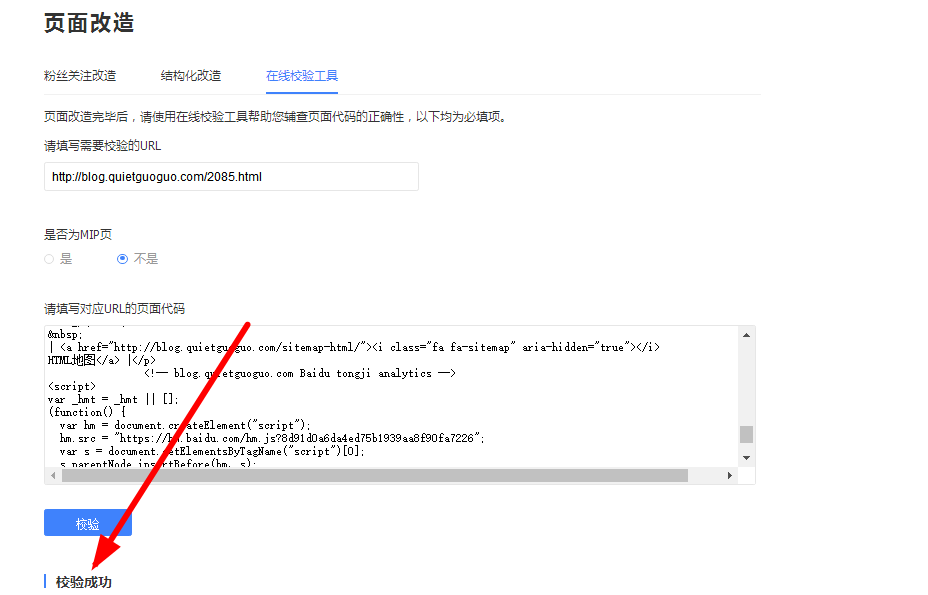<!-- 百度熊掌号页面改造 -->
<script src="//msite.baidu.com/sdk/c.js?appid=你的熊掌号ID"></script>
<?php
if(is_single()){
echo '<script type="application/ld+json">
{
"@context": "https://ziyuan.baidu.com/contexts/cambrian.jsonld",
"appid": "你的熊掌号ID",
"title": "'.get_the_title().'",
"images": ["'.fanly_post_imgs().'"],
"description": "'.fanly_excerpt().'",
"pubDate": "'.get_the_time('Y-m-d\TH:i:s').'"
}
</script>';
}
?>

function.php文件修改

//百度熊掌号页面改造
//获取文章/页面摘要
function fanly_excerpt(\$len=220){
if ( is_single() || is_page() ){
global \$post;
if (\$post->post_excerpt) {
\$excerpt  = \$post->post_excerpt;
} else {
if(preg_match('/<p>(.*)<\/p>/iU',trim(strip_tags(\$post->post_content,"<p>")),\$result)){
\$post_content = \$result['1'];
} else {
\$post_content_r = explode("\n",trim(strip_tags(\$post->post_content)));
\$post_content = \$post_content_r['0'];
}
\$excerpt = preg_replace('#^(?:[\x00-\x7F]|[\xC0-\xFF][\x80-\xBF]+){0,0}'.'((?:[\x00-\x7F]|[\xC0-\xFF][\x80-\xBF]+){0,'.\$len.'}).*#s','\$1',\$post_content);
}
return str_replace(array("\r\n", "\r", "\n"), "", \$excerpt);
}
}
//优先获取文章中的三张图，否则依次获取自定义图片/特色缩略图/文章首图 last update 2017/11/23
function fanly_post_imgs(){
global \$post;
\$content = \$post->post_content;
preg_match_all('/<img .*?src=[\"|\'](.+?)[\"|\'].*?>/', \$content, \$strResult, PREG_PATTERN_ORDER);
\$n = count(\$strResult);
if(\$n >= 3){
\$src = \$strResult.'","'.\$strResult.'","'.\$strResult;
}else{
if( \$values = get_post_custom_values("thumb") ) {	//输出自定义域图片地址
\$values = get_post_custom_values("thumb");
\$src = \$values ;
} elseif( has_post_thumbnail() ){	//如果有特色缩略图，则输出缩略图地址
\$thumbnail_src = wp_get_attachment_image_src(get_post_thumbnail_id(\$post->ID),'full');
\$src = \$thumbnail_src ;
} else {	//文章中获取
if(\$n > 0){ // 提取首图
\$src = \$strResult;
}
}
}
return \$src;
}

single.php文件修改

<script>cambrian.render('body')</script>
<script>cambrian.render('tail')</script>在线校验